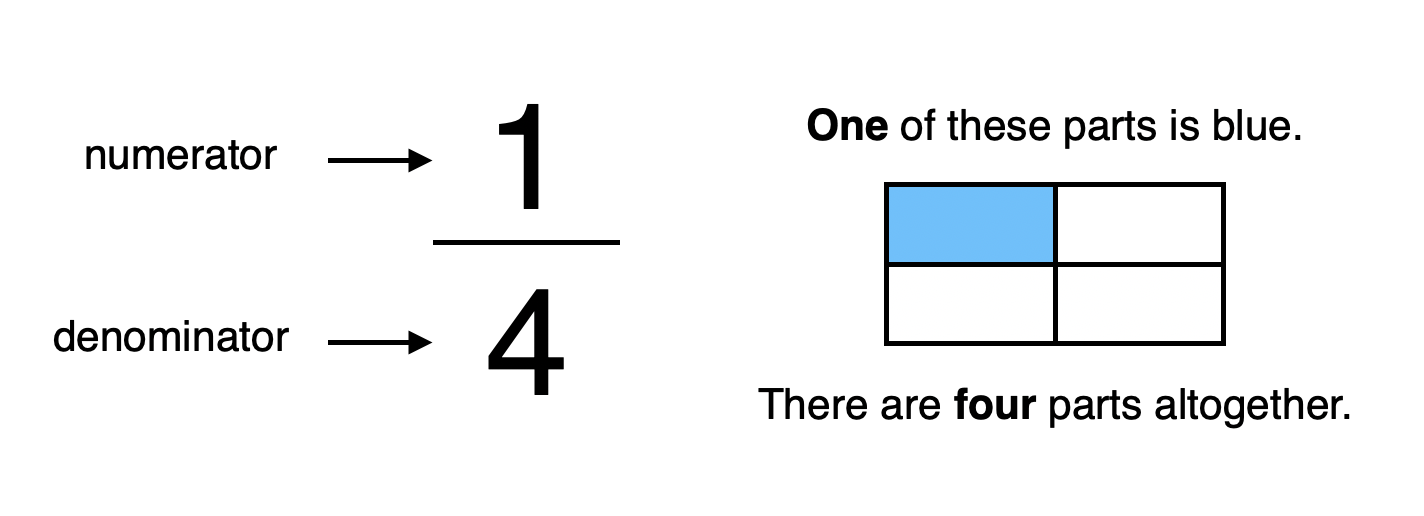# Fractions of Amounts

## What is a fraction?

A fraction is a part (or parts) of something.

A fraction is written like this:The numerator shows us how many parts of something we have.

The denominator shows us how many parts there are in total.

## Finding fractions of amounts

To find a fraction of an amount, we have to work out how much that part is worth within the whole.

There are different ways to do this:

Visual grouping

Let's look at how to find 1/4 of 20:

1. Look at the denominator (4). It tells you how many groups you need to share the amount (20) into equally.

2. Draw four circles to represent the groups. Share the amount (20) into them equally by drawing dots, crosses, etc.

3. Look at the numerator (1).   It tells you how many of the groups you need to look at.

4. There are 5 dots in one group.

So, 1/4 of 20 = 5

Using multiplication and division

Let's look at how to find 3/4 of 20:

1. Divide the amount (20) by the denominator (4).

2. Multiply this answer by the numerator (3).

So, 3/4 of 20 = 15

This method can also be used to find out a fraction of a set of objects, or a fraction of a shape:

## Fractions of amounts KS1

Finding fractions of amounts is first mentioned in Year 2 of the Mathematics Programme of Study in the National Curriculum:

## Fractions of amounts Year 2:

• write simple fractions for example, 1/2 of 6 = 3 and recognise the equivalence of 2/4 and 1/2.

This objective is covered in our Year 2 scheme, 'Can we find fractions of numbers?'

## Fractions of amounts KS2

Finding fractions of amounts is specifically mentioned in Years 3 and 4 of the Mathematics Programme of Study in the National Curriculum:

## Fractions of amounts Year 3:

• recognise, find and write fractions of a discrete set of objects: unit fractions and non-unit fractions with small denominators

This objective is covered in our Year 3 scheme, 'Finding Fractions'.

## Fractions of amounts Year 4:

• solve problems involving increasingly harder fractions to calculate quantities, and fractions to divide quantities, including non-unit fractions where the answer is a whole number

This objective is covered in our Year 4 scheme, 'Proportion Problems'.